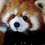# Some intuition behind the prime number theorem

It's generally easy to think about difficult theorems on prime numbers in terms of non-rigorous heuristics. Relatively simple statements, such as the twin prime conjecture, Dirichlet's Theorem, and the Prime Number Theorem, turn out to be very hard to prove. Really hard to prove, that is. The first is still a conjecture, as the name implies, while we still don't exactly know the error term on the third (the Riemann Hypothesis, if true, will tell us what it is). The unifying theme behind them is that they all have relatively simple arguments in their favor.

Here, I'll focus on a non-rigorous justification of the prime number theorem. Take the function $\pi (x)$ to represent the number of prime numbers below $x$. For example, $\pi (10) = 4 , \: \pi (100) = 25, \: \pi (100,000) = 9,592$. The prime number theorem tells us that, asymptotically, the prime-counting function behaves as.

$\pi(x) \simeq \frac{x}{lnx}$

To "prove" this, let's start with the oldest algorithm for finding prime numbers: the Sieve of Eratosthenes. We start with the number $2$ and "cross out" all of its multiples, which leaves $1/2$ of all numbers marked and half of them unmarked. From those remaining non-multiples, we move to the next number ($3$ in this case) and do the same, leaving $2/3$ of the remaining numbers unmarked. We move on to $5$, leaving $4/5$ numbers unmarked, et cetera.

Here's an animation of the sieve in action.These unmarked numbers are all potential primes. As the sieve continues, it will continue finding new primes while narrowing down the candidates for potential primes. Intuitively, this also means that the density of primes decreases at high values of $n$. Up to a number $n$, the fraction of unmarked numbers along the entire set of integers is

$\displaystyle \prod_{p

There are two important observations to make here: The first is that this product converges to $0$ as $n \rightarrow \infty$, which means that prime numbers have zero density with respect to integers. This makes sense, since the sieve can only take away prime candidates. Thus, the density of prime numbers can only decrease. In other words, $\pi (n) / n \rightarrow 0$ when $n \rightarrow \infty$.

Second, you can cut off the number line above $n$ to give the local density of primes. This means that

$\frac{\pi (n)}{n} \approx \displaystyle \prod_{p

Since this goes to $0$, its asymptotic behavior doesn't seem that exciting, but we can look at how exactly it approaches $0$ by seeing how its reciprocal diverges. We have

$\frac{n}{\pi (n)} \approx \displaystyle \prod_{p

Using the taylor series for $\frac{1}{1-x}$, we can turn this into

$\frac{n}{\pi (n)} \approx \displaystyle \prod_{p

Where $N$ is the set of all integers with prime factors less than $n$. This sum itself is difficult to evaluate, so this is where we need to turn to hand-waviness. We can split the sum up into two parts

$\displaystyle \sum_{k \in N} \frac{1}{k} = \displaystyle \sum_{k \le n}^n \frac{1}{k} + \displaystyle \sum_{k \in N,k>n }^\infty \frac{1}{k} \approx \displaystyle \sum_{k \le n}^n \frac{1}{k}$

Where the last term was ignored because it's more or less smaller than the former. Thankfully, we at least know how to evaluate this sum. Note that this sum is essentially a Riemann integral of $f(x) = 1/x$, so its asymptotic behavior is essentially its antiderivative, or $ln(x)$.Thus, we have:

$\frac{n}{\pi (n)} \approx \displaystyle \sum_{k \le n}^n \frac{1}{k} \simeq ln(n)$

Rearranging stuff gives us the answer that we were after:

$\large \pi (n) \simeq \frac{n}{ln(n)}$

The density observed earlier thus falls off asymptotically as:

$\displaystyle \prod_{p

To me, this is a pretty natural argument in favor of the prime number theorem. While it requires a lot of work from complex analysis to prove rigorously, this is still the most intuitive approach to me.Note by Levi Walker
2 years, 5 months ago

This discussion board is a place to discuss our Daily Challenges and the math and science related to those challenges. Explanations are more than just a solution — they should explain the steps and thinking strategies that you used to obtain the solution. Comments should further the discussion of math and science.

When posting on Brilliant:

• Use the emojis to react to an explanation, whether you're congratulating a job well done , or just really confused .
• Ask specific questions about the challenge or the steps in somebody's explanation. Well-posed questions can add a lot to the discussion, but posting "I don't understand!" doesn't help anyone.
• Try to contribute something new to the discussion, whether it is an extension, generalization or other idea related to the challenge.

MarkdownAppears as
*italics* or _italics_ italics
**bold** or __bold__ bold
- bulleted- list
• bulleted
• list
1. numbered2. list
1. numbered
2. list
Note: you must add a full line of space before and after lists for them to show up correctly
paragraph 1paragraph 2

paragraph 1

paragraph 2

[example link](https://brilliant.org)example link
> This is a quote
This is a quote
    # I indented these lines
# 4 spaces, and now they show
# up as a code block.

print "hello world"
# I indented these lines
# 4 spaces, and now they show
# up as a code block.

print "hello world"
MathAppears as
Remember to wrap math in $$ ... $$ or $ ... $ to ensure proper formatting.
2 \times 3 $2 \times 3$
2^{34} $2^{34}$
a_{i-1} $a_{i-1}$
\frac{2}{3} $\frac{2}{3}$
\sqrt{2} $\sqrt{2}$
\sum_{i=1}^3 $\sum_{i=1}^3$
\sin \theta $\sin \theta$
\boxed{123} $\boxed{123}$

Sort by:

WOW!!!! This is pretty great!!!!! I mean, a great way to approach the proof in a not so rigorous way, but still get a fairly good idea about it!!!! :) Also, a small typo, it should be Sieve of "Eratosthenes"........

- 2 years, 5 months ago

I could never spell his name right for some reason :p and thanks! Heuristic arguments like this are probably my favorite parts of mathematics.

- 2 years, 5 months ago